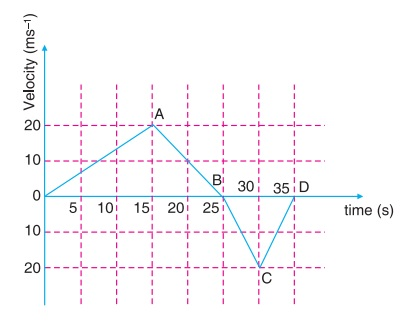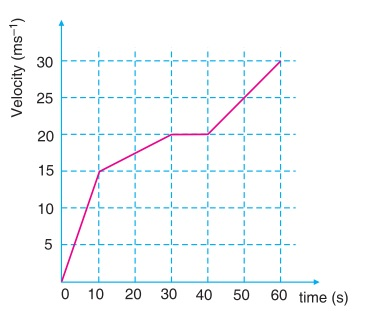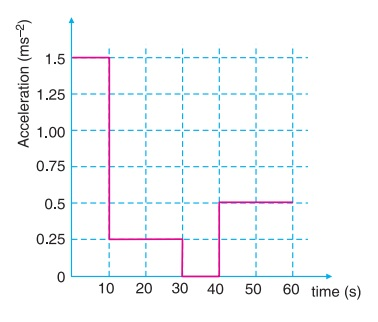# Acceleration

In the non-uniform motion, the change in velocity in different intervals of motion is different. This change in velocity with time is called acceleration.

Thus, the acceleration of an object is defined as the change in velocity divided by the time interval during which this occurs.

Acceleration = Change in velocity / Time interval

Its unit is ms–2. It is specified by direction. Its direction is along the direction of change in velocity.

Example: Suppose the velocity of an object changes from 10 ms–1 to 30 ms–1 in a time interval of 2 s.

Acceleration, a = (30 – 10) ms–1 / 2 s

= 10 ms–2

This means that the object accelerates in +x direction and its velocity increases at a rate of 10 ms–1 in every second.

If the acceleration of an object during its motion is constant, then object is moving with uniform acceleration. The velocity-time graph of such a motion is straight line inclined to the time axis.For a given time interval, if the final velocity is more than the initial velocity, then the acceleration will be positive. However, if the final velocity is less than the initial velocity, the acceleration will be negative.

When velocity of the object is constant, acceleration will be zero. Thus, for uniform motion, the acceleration is zero and for non-uniform motion, the acceleration is nonzero.

Example 1: Find the distance and displacement from the given velocity-time graph.Distance travelled = Area of ΔOAB + Area of ΔBCD

= ½ × (25) × (20) + ½ × (10) × (20)

= 250 + 100 = 350 m

Displacement = Area of ΔOAB – Area of ΔBCD

= 250 – 100 = 150 m

Example 2: From the given velocity-time graph obtain the acceleration-time graph.From the given graph acceleration for 0 – 10 s time interval

= (15 - 0) / (10 - 0) = 1.5 ms–2

Acceleration for 10 – 20 s time interval in same as for 20 – 30 s time interval

= (20 - 15) / (30 - 10) = 0.25 ms–2

Acceleration for 30 – 40 s time interval

= (20 - 20) / (40 - 30) = 0

Acceleration for 40 – 50 and 50 – 60 s interval

= (30 - 20) / (60 - 40) = 0.5 ms–2

For all the above time intervals the acceleration-time graph can be drawn as: